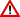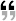# What is Category Theory?I wrote this about four years ago, and my understanding of category theory has advanced hugely since then. Take this with a grain of salt. None of it is wrong, but in hindsight it looks a bit silly.

 This is my attempt at explaining the point of Category Theory. The idea is to give an idea of why one might want to invest time in understanding the concepts in this area, rather than to properly explain the concepts themselves.

Let's suppose you've decided that you want to study functions. Let's also suppose you want to do so in a really, really general manner.

What can you do with functions? Well, you can apply a function, but let's forget about that for a moment and consider everything else.

• Let's assume we know what we mean when we say that two functions are equal, and that this equality is an equivalence relation.

• Every function has a domain and a range. We won't say what these are (for example, we're not going to assume they are sets!), but we'll assume that we know when they match up.

• If you have two functions – and the domain of one matches the range of the other – we'll assume that you can compose them to make a new function.

• If you compose three functions, it shouldn't matter in what order you perform the compositions. In other words, composition should be associative: ((f o g) o h)=(f o (g o h)), where the “=” sign is that equality we mentioned earlier. Notice that we defined what we mean by “associative” without having to talk about actually applying the functions – this will be a recurring theme.

• For every domain or range, we ought to be able to cook up an identity function on it. Again, we need to define “identity” without talking about applying functions, so we'll say that i is an identity if for all f, (f o i)=f and (i o f)=f. Note again that we defined what we mean by “identity” without having to talk about applying the function.

Category Theory is the study of function-like things that have all of the above properties (and perhaps more, but we ignore them). Essentially, the entirety of category theory is the answer to a single question: “assuming we're dealing with function-like things with the properties above, what can we prove about them using only statements phrased in terms of equality, composition, and identity?”

I say “function-like” (the technical term is “morphism”) because category theory can be applied to any collection of things with the properties above, even if they aren't functions! For example relations aren't always functions, but they happen to have all of the above properties, so you can study non-function relations using category theory. Likewise, we can apply category theory to the study of a particular kind of function, as long as there are identities of that kind and as long as that kind is closed under composition. Isomorphisms of groups are one example – not all functions are isomorphisms of groups, but there is an identity isomorphism for every group, and composing two isomorphisms always gives you back an isomorphism.

Everything above could just be considered a really verbose and less-sophisticated rephrasing of the following:What we are probably seeking is a “purer” view of functions: a theory of functions in themselves, not a theory of functions derived from sets. What then is a pure theory of functions? Answer: category theory. – Dana Scott, Relating Theories of the Lambda-Calculus. (1980)

## Recovering Element-Based Concepts

Now let's go back to the “concrete” case where we're really talking about sets and functions. Consider the set 2, which has two elements; we'll call one of them true and the other false.

Given some other set X, how many functions are there from X to 2? Clearly there are 2|X| such functions. How many subsets of X are there? There are 2|X| subsets! Is this a coincidence? No – in fact, for every subset of X there is a function which sends exactly those values to true, and for every function from X to 2 there is a subset of X consisting only of the elements sent to true. So, here is the kicker: a choice of function from X to 2 holds exactly as much information as a choice of a subset of X. Whenever we used to speak of subsets of X, we can instead speak of functions from X to 2 with no loss of specificity.

The beauty of this concept is that the choice of a function (or “morphism”) does not require talking about elements – so it fits within category theory and lets us talk about “subsets” even when we're not sure if the things we're talking about are “sets” (or even “have elements”!). Granted, we assumed that there was some object 2 that “has two elements”, but it turns out there is a way to say “acts as if it had two elements for all category-theoretic purposes”.

There are many more examples of this phenomenon: the “elements” of X – or the analogs thereof – correspond to the functions from 1 to X where 1 is any one-element set. The 0 object (empty set) has the following properties that can be stated within category theory: there are no functions to it from any other set except itself, and a single unique function from it to every other set. The 1 object has a unique function to it from every other set.

One of the most difficult and most powerful parts of category theory is rebuilding all of the “element-based” concepts we're used to from set theory using only morphisms. It's really amazing how many of these concepts can be rebuilt!

## Relationship to Set Theory

It seems to be an unstated assumption among mathematical logicians that first-order logic is above reproach as a foundational ingredient (this appears to include the equality relation, law of excluded middle, and infinite collections of axioms).

One of the particularly interesting aspects of category theory is that none of the assumptions we've made is phrased in terms of anything beyond first-order logic – not even naive set theory. This makes category theory an interesting and useful vehicle for exploring things that might not fit into ZF very nicely, as long as they can be described in terms of function-like things rather than sets.

Indeed, a “syntactically” motivated person might say that ZF set theory is just the study of the behavior of a left-extensional, well-founded two-place relation in first-order logic. In fact, according to the Mostowski's Collapse Lemma ZFC is the most general such algebra which obeys limitation-of-size. All the other axioms of ZFC simply assert that elements (sets) satisfying various relations must exist. Such a person would similarly say that category theory is the study of the behavior – in first order logic – of an associative two-place partial function (or three-place relation) which is left- and right-extensional on the elements designated as “identity morphisms”.

## Relationship to Type Theorywork in progress; may contain inaccuracies

Typically a programming language is viewed as a category whose objects are the types of the language and whose (expressible) functions are the morphisms between those types. This framework can be used to study type constructors such as List, which take any type X to the type List(X) – even if X is List(Y) for some Y.

From this standpoint, type constructors like List are functors from the programming-language-category to itself. That doesn't say much if you're only looking at what the functor does to types – it just says that the functor maps types to types, so if X is a type then List(X) is a type. Nothing earth-shattering there.

The real insights come from looking at what the functor rules for morphisms mean in the context of typed programming languages. Because functors map not only objects but also morphisms, in order to look at List(X) as a functor we need to come up with a way to turn functions on X's into functions on List(X)'s in a way that obeys the functor-laws. It turns out that the map functional is exactly what we're looking for, and that the functor laws correspond to some fairly useful properties that the map function has – one of them being a fusion law.

The big win comes from generalization: there are many other type constructors that act as functors, and by looking at them as functors we can in one fell swoop crank out a whole family of facts about the map-like operator they define for bringing functions.

The Haskell Functor type class partially formalizes this; an instance Functor (F t) indicates that F maps types to types (again, no big surprise), but also indicates that for a specific type t, the functor will provide a function fmap that takes t-functions to (F t)-functions. The only thing missing is the proof that fmap complies with the functor laws, but that cannot be expressed without a more sophisticated (probably dependent) type system.

## Application to Functional Programmingwork in progress; may contain inaccuracies

Aside from studying the types of functional programming languages, a category-theoretic or implicit viewpoint is also useful for studying the terms of functional programming languages – even those which are untyped.

Briefly, we can define the explicit study of functions to be the behavior of particular lambda-terms under beta-reduction. We can describe properties of various programs by proving some proposition quantified over all possible arguments to a particular lambda-term.

By contrast, the implicit approach focuses on universal properties – talking about functions using only the composition operator and function names rather than function application and explicit lambda-definition. From this approach, most properties take the form of equalities in the language of functions with the composition symbol.

## Application to Automatawork in progress; may contain inaccuracies

An automaton is generally specified by:

1. A collection of states

2. A collection of inputs

3. A collection of outputs

4. A map from (state,input) pairs to outputs

5. A map from (state,input) pairs to [new] states

Note that, viewed from this perspective, an automaton is actually an algebra in the sense of General Algebra.

The collections mentioned in the first three points could vary widely; for example, finite automata use finite sets while other kinds of automata use modules or topological spaces. The maps mentioned in the last two points might be limited to only particular kinds of functions (for example, continuous functions on topological spaces), might be functions with extra information (for example, a probability distribution in stochastic automata) or might not be functions at all (for example, nondeterministic automata use relations rather than functions, partial automata use partial functions).

By working in the more general framework of category theory – rather than the specific case of sets and functions – it is possible to arrive at results about automata that can be applied generally to all sorts of automata rather than only those which use a particular kind of collection or map. Often such results take the form of equivalences between various automata, particularly when one automaton has fewer states or even a minimal set of states.

The book Universal Theory of Automata: A Categorical Approach, ISBN 3519020548, edited by Ehrig, Kiermeier, Kreowski, and Kuhnel, goes into all this in far greater detail.

## Topoi

There's a good “what are topoi” article here